Next: Missed branches Up: Implementation Previous: Procedure for following branches   Contents

Full continuation procedure

This procedure will determine initial points on the branches of the system for the value of the parameter within some bounds and then follow the branches. It is implemented as

int ALIAS_Full_Continuation(int m,int n,
INTERVAL_VECTOR (* IntervalFunction)(int,int,INTERVAL_VECTOR &),
INTERVAL_MATRIX (* IntervalHessian)(int,int,INTERVAL_VECTOR &),
INTERVAL_VECTOR &Domain,
int M,
double epsilon,double epsilonf,
double *z,double delta,double mindelta,double mindz,
INTERVAL &Rangez,
int sens,
MATRIX &BRANCH,int *NBBRANCH)
The arguments are the same than for the previous procedure except for:
• Domain: the range for the m variable in which we will look for solutions of the system,
• M is the maximum number of boxes which may be stored (see the note 2.3.4.5)
• mindz: the accuracy with which the starting point for the branches will be determined: if the value of the parameter at which the branches will start is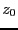, then the system has no solution for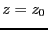-mindz. This is done by using a bisection on the parameter: if at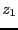the system has no solution and has a positive number of solution at+delta, then we will solve the system for+delta/2. At each time we will store the value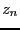of the parameter for which there is no solution of the system and the value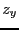for which we have solutions and we will stop the bisection on the parameter as soon as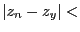mindz.
To determine the starting points of the branches this procedure uses the solving procedure with the Hessian (see section 2.5). As soon as initial valid solutions are found the ALIAS_Start_Continuation procedure is called until either a bound of the parameter range is reached, or a singularity occur. In the later case the solving procedure is called with an increased value for the parameter. This algorithm cannot find isolated points and may miss branches (see next section).

There is also another version of this program where you indicate just before Domain the solutions which have already been found. The syntax is

INTERVAL_MATRIX (* IntervalHessian)(int,int,INTERVAL_VECTOR &),
int NUM,
INTERVAL_MATRIX &Solutions,
INTERVAL_VECTOR &Domain

The return code for these procedures are:

•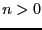: the number of branches found by the algorithm
• -1: no initial point have been found
• -10: Newton algorithm has failed (should not occur)
• -20: sens is not 1 or -1
• -30: delta or mindelta is negative

Finding the initial starting point with the accuracy mindz may be computer intensive. Hence the integer global variable ALIAS_Allow_Backtrack enable to disable this process if it is set to 0 (its default value is 1): in that case as soon as starting points have been found (hence at+delta) we will start following the branches.

In fact these procedures are special occurrences of another ALIAS procedure which has another argument right after the Hessian argument. Assume for example that you are considering a system which has one equation written as: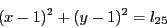where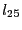is the parameter of the system and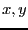the unknowns. When using the continuation method we have to define ranges for these unknowns in order to be able to solve the system of equations. Up to now we have indicated bounds that are constants but for the equation example it will be interesting to be able to specify that these bounds may change according to the value for the parameter using a simplification procedure. In our example clearly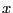andcannot exceed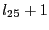and cannot be lower than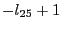(this is an application of the concept of 2B-consistency, see section 2.17). Hence we may specify right after the Hessian argument the name of a procedure, for example Range, that is able to update the bounds on the unknowns according to the value of the parameter (or any other variable that may play a role). The syntax of the procedure Range is:
INTERVAL_VECTOR Range(double z, INTERVAL_VECTOR &Variable)
where z is parameter value and Variable the current set of ranges for the unknowns. This procedure must return a set of ranges for the unknowns (be careful to check that the returned ranges are included in the initial ranges).

Note also that the ALIAS-Maple package offers a procedure that uses the method described in section 2.14 for finding the initial starting points of the branches: this method is efficient if the equations include linear terms in the unknowns.Next: Missed branches Up: Implementation Previous: Procedure for following branches   Contents
Jean-Pierre Merlet 2012-12-20